Tutorials
python

# Introduction To GUI With Tkinter In Python

In this tutorial, you are going to learn how to create GUI apps in Python. You'll also learn about all the elements needed to develop GUI apps in Python.

Hi, Welcome to your first Graphical User Interface(GUI) tutorial with Tkinter in Python. In this tutorial, you will learn how to create GUI apps in Python using the Tkinter module.

You should be familiar with the Python to learn Tkinter. If you don't know how to code in Python, I recommend you to take this free Datacamp Python Course.

You can also read the Python Official Documentation if you want.

6.1 Grid

9. Classes

15. EndNote

## 1. What Is A Graphical User Interface(GUI)

GUI is a desktop app which helps you to interact with the computers. They are used to perform different tasks in the desktops, laptops, other electronic devices, etc.., Here, we mainly talking about the laptops and desktops.

• GUI apps like Text-Editors are used to create, read, update and delete different types of files.
• GUI apps like Sudoku, Chess, Solitaire, etc.., are games which you can play.
• GUI apps like Chrome, Firefox, Microsoft Edge, etc.., are used to surf the Internet.

They are some different types of GUI apps which we daily use on the laptops or desktops. We are going to learn how to create those type of apps.

As this is an Introduction to GUI, we will create a simple Calculator GUI app.

## 2. What Is Tkinter

Tkinter is an inbuilt Python module used to create simple GUI apps. It is the most commonly used module for GUI apps in the Python.

You don't need to worry about installation of the Tkinter module as it comes with Python default.

Note:-

• I am going to use Python 3.6 version. So, kindly update Python if you're using below versions.
• Follow this tutorial along with the practice so, that you can understand it very quickly.
• Don't copy the code. Try to write by modifying it as you like.

## 3. Introduction To Tkinter

Run the following code to create a simple window with the text Hello World!.

Steps:-

• import the module tkinter.
• Initialize the window manager with the tkinter.Tk() method and assign it to a variable window. This method creates a blank window with close, maximize and minimize buttons.
• Rename the title of the window as you like with the window.title(title_of_the_window).
• Label is used to insert some objects into the window. Here, we are adding a Label with some text.
• pack() attribute of the widget is used to display the widget in a size it requires.
• Finally, the mainloop() method to display the window until you manually close it.
import tkinter

window = tkinter.Tk()
# to rename the title of the window
window.title("GUI")
# pack is used to show the object in the window
label = tkinter.Label(window, text = "Hello World!").pack()
window.mainloop()


That's a basic program to create a simple GUI interface. You will see a similar window like this.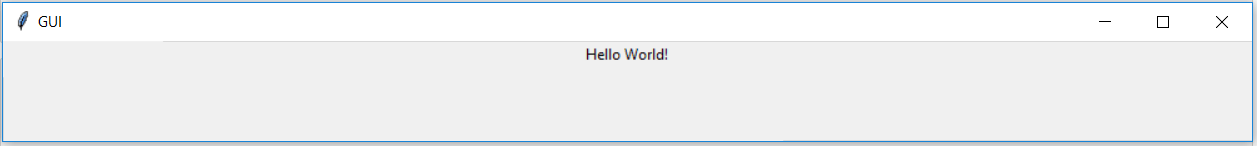## 4. Tkinter Widgets

Widgets are something like elements in the HTML. You will find different types of widgets to the different types of elements in the Tkinter.

Let's see the brief introduction to all of these widgets in the Tkinter.

• Button:- Button widget is used to place the buttons in the tkinter.
• Canvas:- Canvas is used to draw shapes in your GUI.
• Checkbutton:- Checkbutton is used to create the check buttons in your application. You can select more than one option at a time.
• Entry:- Entry widget is used to create input fields in the GUI.
• Frame:- Frame is used as containers in the tkinter.
• Label:- Label is used to create a single line widgets like text, images, etc..,

I mentioned only some of the widgets that are present in Tkinter. You can find the complete list of widgets at official Python documentation.

## 5. Geometry Management

All widgets in the tkinter will have some geometry measurements. These measurements give you to organize the widgets and their parent frames, windows, etc..,

Tkinter has the following three Geometry Manager classes.

• pack():- It organizes the widgets in the block, which mean it occupies the entire available width. It's a standard method to show the widgets in the window
• grid():- It organizes the widgets in table-like structure. You will see details about grid later in this tutorial.
• place():- It's used to place the widgets at a specific position you want.

## 6. Organizing Layout And Widgets

To arrange the layout in the window, we will use Frame, class. Let's create a simple program to see how the Frame works.

Steps:-

• Frame is used to create the divisions in the window. You can align the frames as you like with side parameter of pack() method.
• Button is used to create a button in the window. It takes several parameters like text(Value of the Button), fg(Color of the text), bg(Background color), etc..,

Note:- The parameter of any widget method must be where to place the widget. In the below code, we use to place in the window, top_frame, bottom_frame.

import tkinter

window = tkinter.Tk()
window.title("GUI")

# creating 2 frames TOP and BOTTOM
top_frame = tkinter.Frame(window).pack()
bottom_frame = tkinter.Frame(window).pack(side = "bottom")

# now, create some widgets in the top_frame and bottom_frame
btn1 = tkinter.Button(top_frame, text = "Button1", fg = "red").pack()# 'fg - foreground' is used to color the contents
btn2 = tkinter.Button(top_frame, text = "Button2", fg = "green").pack()# 'text' is used to write the text on the Button
btn3 = tkinter.Button(bottom_frame, text = "Button2", fg = "purple").pack(side = "left")# 'side' is used to align the widgets
btn4 = tkinter.Button(bottom_frame, text = "Button2", fg = "orange").pack(side = "left")

window.mainloop()


Above code produces the following window, if you didn't change the above code.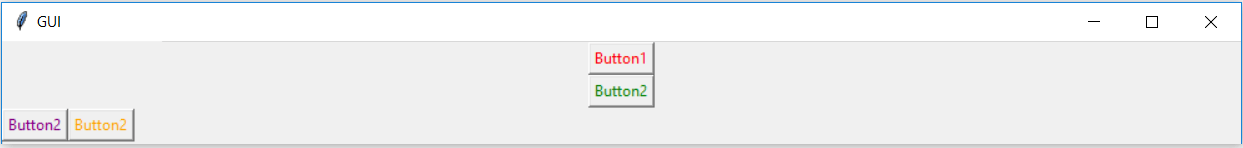Now, we will see how to use the fill parameter of pack()

import tkinter

window = tkinter.Tk()
window.title("GUI")

# creating 3 simple Labels containing any text

# sufficient width
tkinter.Label(window, text = "Suf. width", fg = "white", bg = "purple").pack()

# width of X
tkinter.Label(window, text = "Taking all available X width", fg = "white", bg = "green").pack(fill = "x")

# height of Y
tkinter.Label(window, text = "Taking all available Y height", fg = "white", bg = "black").pack(side = "left", fill = "y")

window.mainloop()


If you run the above code, you will get the output something similar to the following.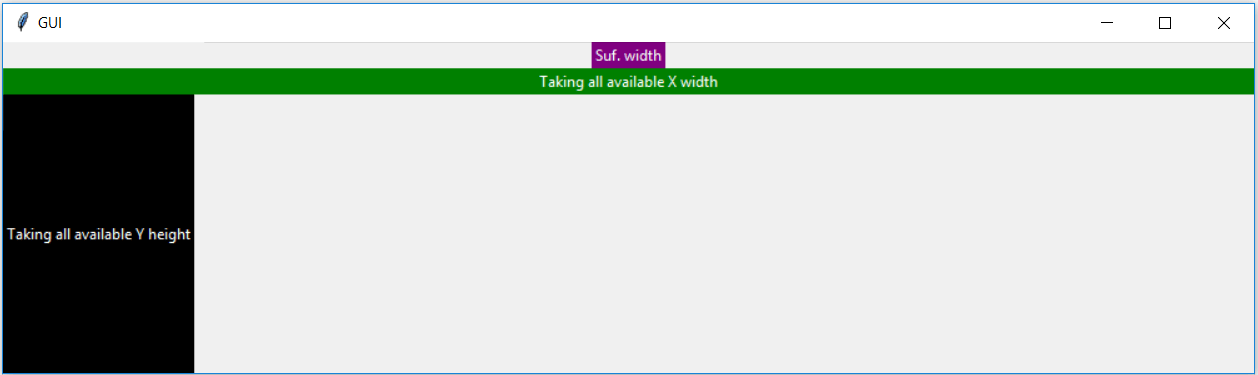### 6.1. Grid

Grid is another way to organize the widgets. It uses the Matrix row column concepts. Something like this.

2 x 2 Matrix

0 0     0 1

1 0     1 1


See the below example to get an idea of how it works.

import tkinter

window = tkinter.Tk()
window.title("GUI")

# creating 2 text labels and input labels

tkinter.Label(window, text = "Username").grid(row = 0) # this is placed in 0 0
# 'Entry' is used to display the input-field
tkinter.Entry(window).grid(row = 0, column = 1) # this is placed in 0 1

tkinter.Label(window, text = "Password").grid(row = 1) # this is placed in 1 0
tkinter.Entry(window).grid(row = 1, column = 1) # this is placed in 1 1

# 'Checkbutton' is used to create the check buttons
tkinter.Checkbutton(window, text = "Keep Me Logged In").grid(columnspan = 2) # 'columnspan' tells to take the width of 2 columns
# you can also use 'rowspan' in the similar manner

window.mainloop()


You will get the following output.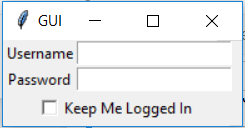## 7. Binding Functions

Calling functions whenever an event occurs refers to a binding function.

• In the below example, when you click the button, it calls a function called say_hi.
• Function say_hi creates a new label with the text Hi.
import tkinter

window = tkinter.Tk()
window.title("GUI")

# creating a function called say_hi()
def say_hi():
tkinter.Label(window, text = "Hi").pack()

tkinter.Button(window, text = "Click Me!", command = say_hi).pack() # 'command' is executed when you click the button
# in this above case we're calling the function 'say_hi'.
window.mainloop()


The above program will produce the following results.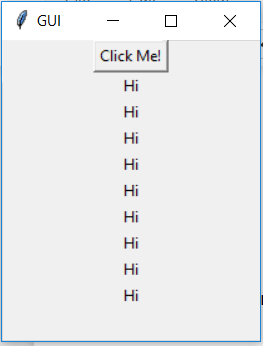Another way to bind functions is using events. Events are something like mousemove, mouseover, clicking, scrolling, etc..,.

The following program also produces the same output as the above one.

• '<Button-1>' parameter of bind method is the left clicking event, i.e., when you click the left button the bind method call the function say_hi

• <Button-1> for left click

• <Button-2> for middle click

• <Button-3> for right click

• Here, we are binding the left click event to a button. You can bind it to any other widget you want.

• You will have different parameters for different events

Let's see

import tkinter

window = tkinter.Tk()
window.title("GUI")

# creating a function with an arguments 'event'
def say_hi(event): # you can rename 'event' to anything you want
tkinter.Label(window, text = "Hi").pack()

btn = tkinter.Button(window, text = "Click Me!")
btn.bind("<Button-1>", say_hi) # 'bind' takes 2 parameters 1st is 'event' 2nd is 'function'
btn.pack()

window.mainloop()


## 8. Mouse Clicking Events

Clicking events are of 3 different types namely leftClick, middleClick, and rightClick.

Now, you will learn how to call a particular function based on the event that occurs.

• Run the following program and click the left, middle, right buttons to calls a specific function.
• That function will create a new label with the mentioned text.
import tkinter

window = tkinter.Tk()
window.title("GUI")

#creating 3 different functions for 3 events
def left_click(event):
tkinter.Label(window, text = "Left Click!").pack()

def middle_click(event):
tkinter.Label(window, text = "Middle Click!").pack()

def right_click(event):
tkinter.Label(window, text = "Right Click!").pack()

window.bind("<Button-1>", left_click)
window.bind("<Button-2>", middle_click)
window.bind("<Button-3>", right_click)

window.mainloop()


If you run the above program, you will see a blank window. Now, click the left, middle and right button to call respective functions.

You get the something similar results to the following.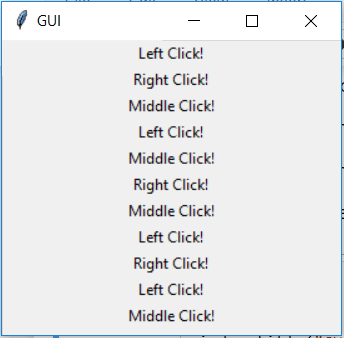## 9. Classes

Classes is handy when you're developing a large software or something that's big.

Let's see how we use Classes in the GUI apps.

import tkinter

class GeeksBro:

def __init__(self, window):

self.text_btn = tkinter.Button(window, text = "Click Me!", command = self.say_hi) # create a button to call a function called 'say_hi'
self.text_btn.pack()

self.close_btn = tkinter.Button(window, text = "Close", command = window.quit) # closing the 'window' when you click the button
self.close_btn.pack()

def say_hi(self):
tkinter.Label(window, text = "Hi").pack()

window = tkinter.Tk()
window.title("GUI")

geeks_bro = GeeksBro(window)

window.mainloop()


The above program produces the output something similar to the following.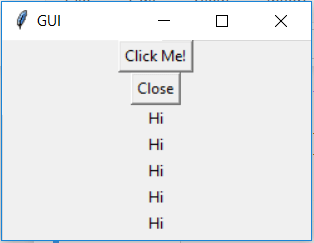I hope all of you know what drop-down menus are. You will create drop-down menus in tkinter using the class Menu. Follow the below steps to create drop-down menus.

Steps:-

• Create a root menu to insert different types of menu options using tkinter.Menu(para) and it takes a parameter where to place the Menu
• You have to tell the tkinter to initiate Menu using window_variable.config(menu = para) and it takes a parameter called menu which is the root menu you previously defined.

Let's see the example to understand it fully.

import tkinter

window = tkinter.Tk()
window.title("GUI")

def function():
pass

file_menu.add_command(label = "New file.....", command = function) # it adds a option to the sub menu 'command' parameter is used to do some action

window.mainloop()


You will see the output something similar to the following. Click to the File and Edit menus to look at the options.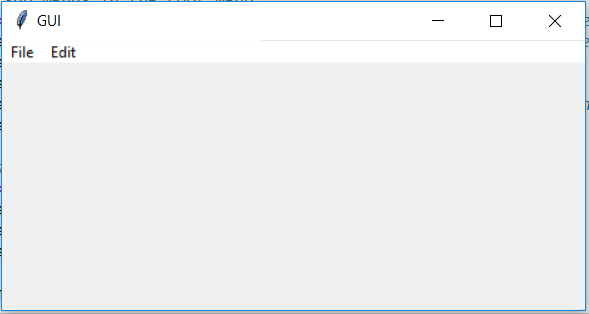You can create alert boxes in the tkinter using messagebox method. You can also create questions using the messasgebox method.

import tkinter
import tkinter.messagebox

window = tkinter.Tk()
window.title("GUI")

# creating a simple alert box
# creating a question to get the response from the user [Yes or No Question]
response = tkinter.messagebox.askquestion("Simple Question", "Do you love Python?")
# If user clicks 'Yes' then it returns 1 else it returns 0
if response == 1:
tkinter.Label(window, text = "You love Python!").pack()
else:
tkinter.Label(window, text = "You don't love Python!").pack()

window.mainloop()


You will see the following output.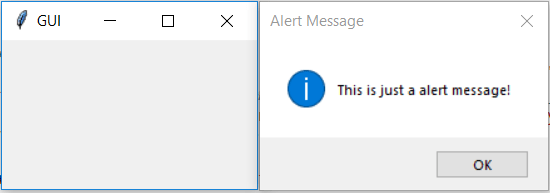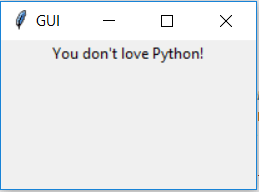## 12. Simple Shapes

You are going to draw some basic shapes with the Canvas provided by tkinter in GUI.

See the example.

import tkinter

window = tkinter.Tk()
window.title("GUI")

# creating the 'Canvas' area of width and height 500px
canvas = tkinter.Canvas(window, width = 500, height = 500)
canvas.pack()

# 'create_line' is used to create a line. Parameters:- (starting x-point, starting y-point, ending x-point, ending y-point)
line1 = canvas.create_line(25, 25, 250, 150)
# parameter:- (fill = color_name)
line2 = canvas.create_line(25, 250, 250, 150, fill = "red")

# 'create_rectangle' is used to create rectangle. Parameters:- (starting x-point, starting y-point, width, height, fill)
# starting point the coordinates of top-left point of rectangle
rect = canvas.create_rectangle(500, 25, 175, 75, fill = "green")

# you 'delete' shapes using delete method passing the name of the variable as parameter.
canvas.delete(line1)
# you 'delete' all the shapes by passing 'ALL' as parameter to the 'delete' method
# canvas.delete(tkinter.ALL)

window.mainloop()


You will see the following shapes in your GUI window.

Just run dir(tkinter.Canvas) to see all the available methods for creating different shapes.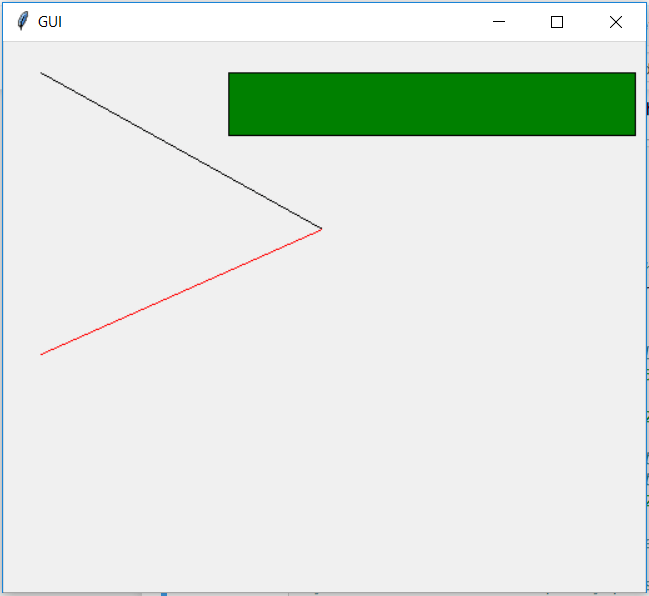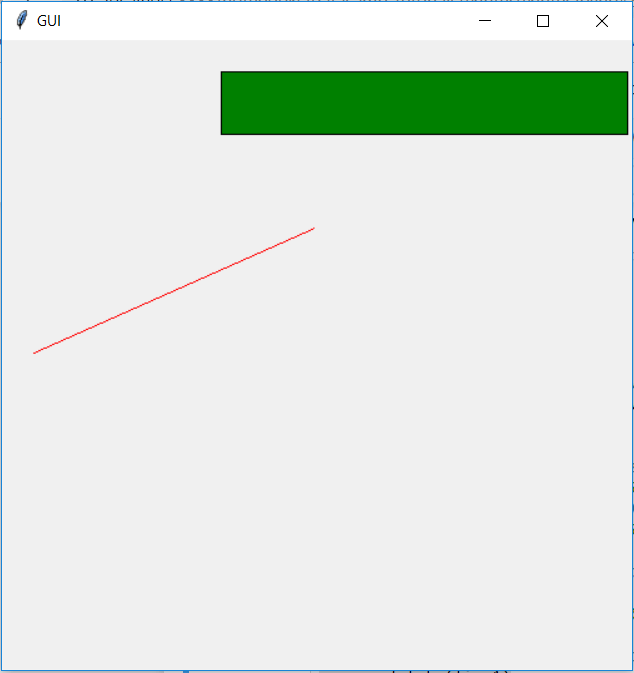## 13. Images And Icons

You can add Images and Icons using PhotoImage method.

Let's how it works.

import tkinter

window = tkinter.Tk()
window.title("GUI")

# taking image from the directory and storing the source in a variable
icon = tkinter.PhotoImage(file = "images/haha.png")
# displaying the picture using a 'Label' by passing the 'picture' variriable to 'image' parameter
label = tkinter.Label(window, image = icon)
label.pack()

window.mainloop()


You can see the icon in the GUI.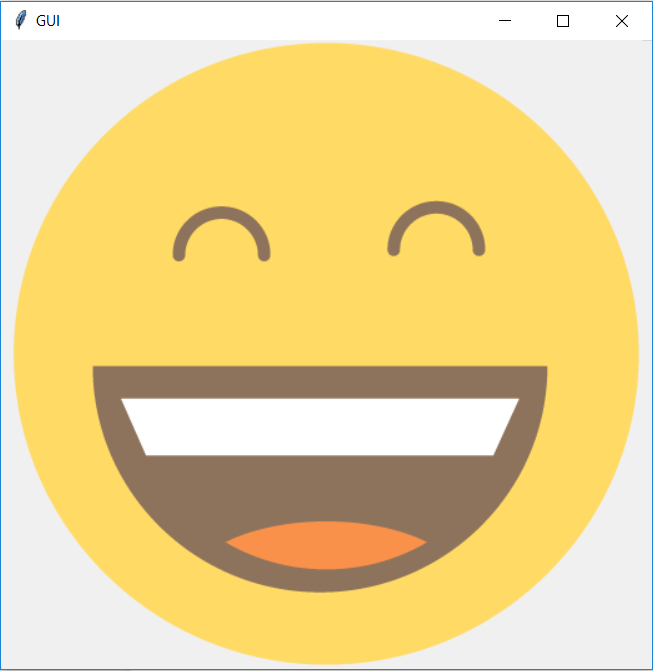Now, you're able to:-

• Understand the tkinter code.
• Create frames, labels, buttons, binding functions, events, etc..,
• To develop simple GUI apps.

Now, we're going to create a simple Calculator GUI with all the stuff you have learned till now.

## 14. Creating Calculator

Every GUI apps include two steps.

• Creating User Interface
• Adding functionalities to the GUI

Let's start creating Calculator.

from tkinter import *

# creating basic window
window = Tk()
window.geometry("312x324") # size of the window width:- 500, height:- 375
window.resizable(0, 0) # this prevents from resizing the window
window.title("Calcualtor")

################################### functions ######################################
# 'btn_click' function continuously updates the input field whenever you enters a number
def btn_click(item):
global expression
expression = expression + str(item)
input_text.set(expression)

# 'btn_clear' function clears the input field
def btn_clear():
global expression
expression = ""
input_text.set("")

# 'btn_equal' calculates the expression present in input field
def btn_equal():
global expression
result = str(eval(expression)) # 'eval' function evalutes the string expression directly
# you can also implement your own function to evalute the expression istead of 'eval' function
input_text.set(result)
expression = ""

expression = ""
# 'StringVar()' is used to get the instance of input field
input_text = StringVar()

# creating a frame for the input field
input_frame = Frame(window, width = 312, height = 50, bd = 0, highlightbackground = "black", highlightcolor = "black", highlightthickness = 1)
input_frame.pack(side = TOP)

# creating a input field inside the 'Frame'
input_field = Entry(input_frame, font = ('arial', 18, 'bold'), textvariable = input_text, width = 50, bg = "#eee", bd = 0, justify = RIGHT)
input_field.grid(row = 0, column = 0)

# creating another 'Frame' for the button below the 'input_frame'
btns_frame = Frame(window, width = 312, height = 272.5, bg = "grey")
btns_frame.pack()

# first row
clear = Button(btns_frame, text = "C", fg = "black", width = 32, height = 3, bd = 0, bg = "#eee", cursor = "hand2", command = lambda: btn_clear()).grid(row = 0, column = 0, columnspan = 3, padx = 1, pady = 1)
divide = Button(btns_frame, text = "/", fg = "black", width = 10, height = 3, bd = 0, bg = "#eee", cursor = "hand2", command = lambda: btn_click("/")).grid(row = 0, column = 3, padx = 1, pady = 1)

# second row
seven = Button(btns_frame, text = "7", fg = "black", width = 10, height = 3, bd = 0, bg = "#fff", cursor = "hand2", command = lambda: btn_click(7)).grid(row = 1, column = 0, padx = 1, pady = 1)
eight = Button(btns_frame, text = "8", fg = "black", width = 10, height = 3, bd = 0, bg = "#fff", cursor = "hand2", command = lambda: btn_click(8)).grid(row = 1, column = 1, padx = 1, pady = 1)
nine = Button(btns_frame, text = "9", fg = "black", width = 10, height = 3, bd = 0, bg = "#fff", cursor = "hand2", command = lambda: btn_click(9)).grid(row = 1, column = 2, padx = 1, pady = 1)
multiply = Button(btns_frame, text = "*", fg = "black", width = 10, height = 3, bd = 0, bg = "#eee", cursor = "hand2", command = lambda: btn_click("*")).grid(row = 1, column = 3, padx = 1, pady = 1)

# third row
four = Button(btns_frame, text = "4", fg = "black", width = 10, height = 3, bd = 0, bg = "#fff", cursor = "hand2", command = lambda: btn_click(4)).grid(row = 2, column = 0, padx = 1, pady = 1)
five = Button(btns_frame, text = "5", fg = "black", width = 10, height = 3, bd = 0, bg = "#fff", cursor = "hand2", command = lambda: btn_click(5)).grid(row = 2, column = 1, padx = 1, pady = 1)
six = Button(btns_frame, text = "6", fg = "black", width = 10, height = 3, bd = 0, bg = "#fff", cursor = "hand2", command = lambda: btn_click(6)).grid(row = 2, column = 2, padx = 1, pady = 1)
minus = Button(btns_frame, text = "-", fg = "black", width = 10, height = 3, bd = 0, bg = "#eee", cursor = "hand2", command = lambda: btn_click("-")).grid(row = 2, column = 3, padx = 1, pady = 1)

# fourth row
one = Button(btns_frame, text = "1", fg = "black", width = 10, height = 3, bd = 0, bg = "#fff", cursor = "hand2", command = lambda: btn_click(1)).grid(row = 3, column = 0, padx = 1, pady = 1)
two = Button(btns_frame, text = "2", fg = "black", width = 10, height = 3, bd = 0, bg = "#fff", cursor = "hand2", command = lambda: btn_click(2)).grid(row = 3, column = 1, padx = 1, pady = 1)
three = Button(btns_frame, text = "3", fg = "black", width = 10, height = 3, bd = 0, bg = "#fff", cursor = "hand2", command = lambda: btn_click(3)).grid(row = 3, column = 2, padx = 1, pady = 1)
plus = Button(btns_frame, text = "+", fg = "black", width = 10, height = 3, bd = 0, bg = "#eee", cursor = "hand2", command = lambda: btn_click("+")).grid(row = 3, column = 3, padx = 1, pady = 1)

# fourth row
zero = Button(btns_frame, text = "0", fg = "black", width = 21, height = 3, bd = 0, bg = "#fff", cursor = "hand2", command = lambda: btn_click(0)).grid(row = 4, column = 0, columnspan = 2, padx = 1, pady = 1)
point = Button(btns_frame, text = ".", fg = "black", width = 10, height = 3, bd = 0, bg = "#eee", cursor = "hand2", command = lambda: btn_click(".")).grid(row = 4, column = 2, padx = 1, pady = 1)
equals = Button(btns_frame, text = "=", fg = "black", width = 10, height = 3, bd = 0, bg = "#eee", cursor = "hand2", command = lambda: btn_equal()).grid(row = 4, column = 3, padx = 1, pady = 1)

window.mainloop()


See the Calculator which you have made. If you have any doubt in the above code, feel free to ask in the comment sections.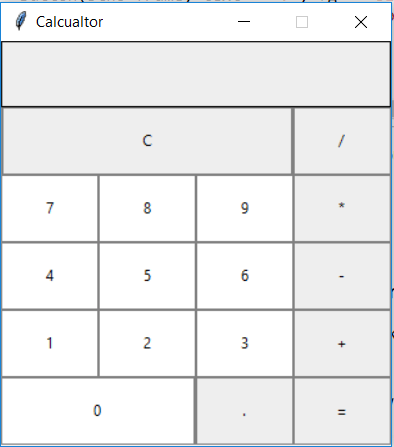## 15. EndNote

This tutorial is not meant to teach you the complete tkinter like documentation. But,

What you have learned in this tutorial is fair enough to make some simple GUI apps. You have to learn more methods for styling and interaction with the objects in GUI.

You can't find the complete documentation of tkinter anywhere. But, learn it by doing and asking someone who knows tkinter well.

Hope you enjoyed this tutorial. Happy Coding!

If you would like to learn more about Python, take DataCamp's Python Data Science Toolbox (Part 1) course.